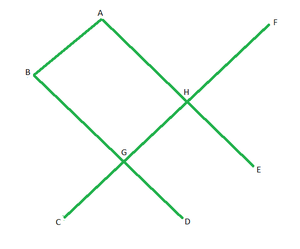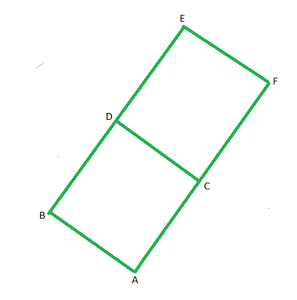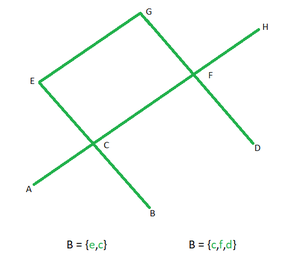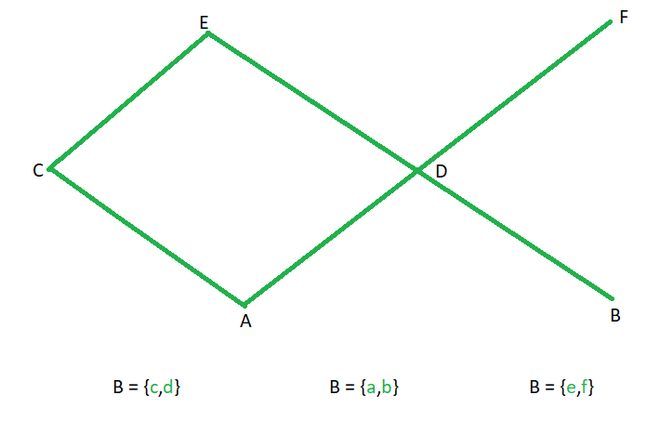# Elements of POSET

Prerequisite: Introduction and Types of Relations

POSET, known as Partially Ordered Set, works on the principle of Partial Ordering Relation. A relation R is said to be Partial Ordered Relation when it can satisfy the following properties:

1. R is Reflexive, i.e., if set A ={1,2,3} then R ={(1,1), (2,2), (3,3)} is a Reflexive relation.
2. R is Anti-symmetric, i.e., if R contains (1,2) then (2,1) is not allowed.
3. R is Transitive, i.e., if R contains (1,2), (2,3), then it should contain (1,3) to make it Transitive.

POSET: If A set ‘A’ following a Partial Ordering Relation ‘R’ then it is known as POSET. It is denoted by [A; R].

Note- Unlike Asymmetry, Anti-Symmetry allows Reflexive elements like (a,a) or (b,b) in a Relation.

Example 1: For a set A = {1,2,3}, check if the following relations are POSET ?

R1= {(1,1), (2,2), (3,3)}

R2= {(1,1), (2,2), (3,3), (1,2), (2,1)}

R3= { }

Explanation: To prove a Partial Order Relation, check Reflexivity, Anti-Symmetry and Transitivity.

R1⇒  Reflexive: Since (1,1) (2,2) (3,3) are present so it is Reflexive.

Anti-symmetry: It allows reflexive pairs, so it is Anti-symmetric.

Transitive: Reflexive pairs are always Transitive.

R2  Reflexive: Since (1,1) (2,2) (3,3) are present so it is Reflexive.

Anti-symmetry: For (1,2) there is (2,1) so not Anti-symmetric.

Transitive: There are no such pairs (a,b) (b,c) such that (a,c) is not present.

R3Reflexive: NULL sets doesn’t contain either of (1,1) (2,2) (3,3).

Therefore, R1 is a POSET, but R2 and R3 are not.

### Elements of POSETElements of POSET

Maximal Element: If in a POSET/Lattice, an element is not related to any other element. Or, in simple words, it is an element with no outgoing (upward) edge. In the above diagram, A, B, F are Maximal elements.

Minimal Element: If in a POSET/Lattice, no element is related to an element. Or, in simple words, it is an element with no incoming (downward) edge. In the above diagram, C, D, E are Minimal elements.Maximum Element (Greatest): If in a POSET/Lattice, it is a Maximal element, and every element is related to it, i.e., every element of the lattice should be connected to this element. In the above diagram, E and F are Maximal elements, but E is the only Maximum element.

Minimum Element (Least): If in a POSET/Lattice, it is a Minimal element and is related to every other element, i.e., it should be connected to every element of the lattice. In the above diagram, A and B are Minimal elements, but A is the only Minimum element.

Note:

• Every Maximum element is a Maximal element but every Maximal element is not a Maximum element
• Every Minimum element is a Minimal element but every Minimal element is not a Minimum element.### Upper Bound

Let’s assume is a subset of set A. An element x ∈ A is in the upper bound of B if (y,x) ∈ POSET where  V y ∈ B. Or we can say that it is an element to which every element of a subset is related.

1. B = {E,C}:     Upper Bound- {G, E}  ( E can itself be an upper bound because Partial order follows Reflexive property)
2. B = {C,F,D}:  Upper Bound- {G, H, F}

### Lower Bound

If B  is a subset of set A, an element x ∈ A is in the lower bound of B if (x,y) ∈ POSET where  V y ∈ B. Or we can say that it is an element that is related /connected to every element of subset B.

1. B = {E,C} :     Lower Bound- {A,B,C}  ( C can itself be a lower bound because Partial order follows Reflexive property)
2. B = {C,F,D} :  Lower Bound- { ∅ }### Least Upper Bound

Also known as Join. The Minimum (Least) element in Upper Bound.

1. B = {C,D} :    Least Upper Bound- { E }
2. B = {A,B} :    Least Upper Bound- { D }
3. B = {E,F}  :    Least Upper Bound- { ∅ }

### Greatest Lower Bound

Also known as Meet. The Maximum (Greatest) element in Lower Bound.

1. B = {C,D} :    Least Upper Bound- { A }
2. B = {A,B} :    Least Upper Bound- { ∅ }
3. B = {E,F}  :    Least Upper Bound- { D }
Whether you're preparing for your first job interview or aiming to upskill in this ever-evolving tech landscape, GeeksforGeeks Courses are your key to success. We provide top-quality content at affordable prices, all geared towards accelerating your growth in a time-bound manner. Join the millions we've already empowered, and we're here to do the same for you. Don't miss out - check it out now!

Previous
Next